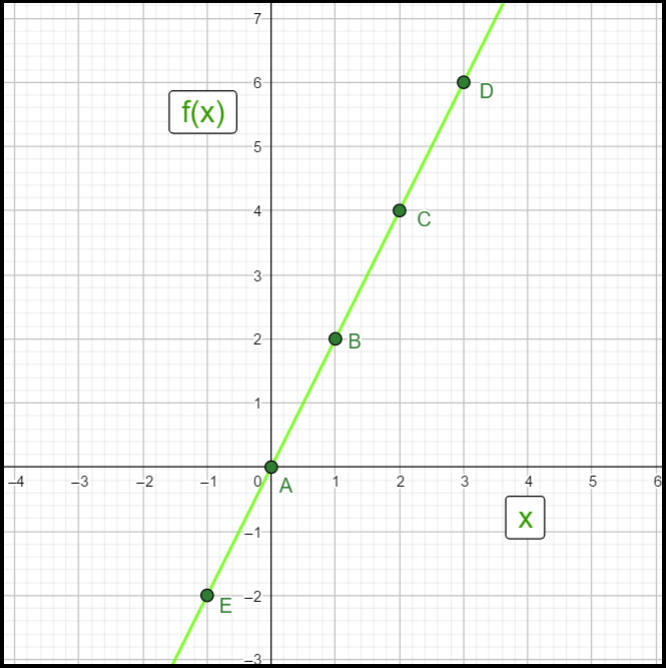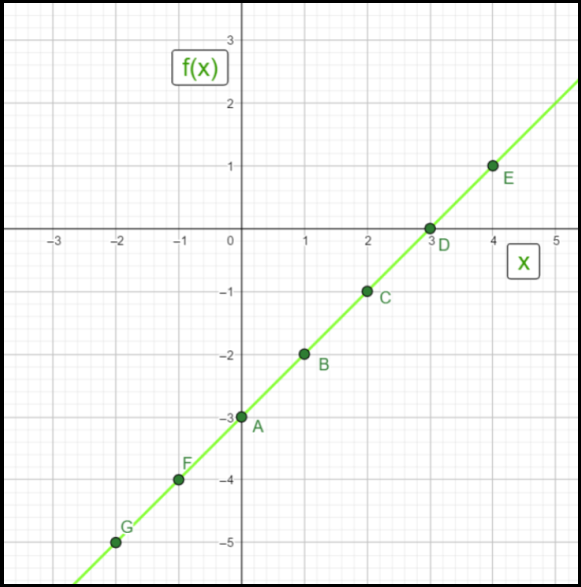Open in App
Not now

# Polynomials in One Variable – Polynomials | Class 9 Maths

• Difficulty Level : Easy
• Last Updated : 27 Oct, 2021

Polynomial originated from the words “poly” which means “many” and the word “nomial” which means “term”. In maths, a polynomial expression consists of variables which are also known as indeterminate and coefficients. Polynomials are expressions with one or more terms with a non-zero coefficient. A polynomial can have more than one term. In the polynomial, each expression in it is called a term.  Suppose x2 + 5x + 2 is polynomial, then the expressions x2, 5x, and 2 are the terms of the polynomial.  Each term of the polynomial has a coefficient. For example, if 2x + 1 is the polynomial, then the coefficient of x is 2. But in this article we are going to discuss the polynomial in one variable. In general,

The algebraic expressions with only one variable are known as Polynomials in one variable.

Examples:

1. P(x) = 4x – 3
2. G(y) = y4 – y2 + 2y + 9

## Degree of Polynomials

The highest exponent of the variable in the algebraic expression is called Degree of a Polynomial.

### Examples

1. P(x) = x3 – 5x2 + 9x + 16     (Degree = 3, because the highest exponent is 3 here)
2. Q(x) = 5      (Degree = 0)

## Evaluating Polynomials

The value of a polynomial at a given value of the variable is termed as evaluation of polynomial.

### Examples

Question 1: Evaluate the polynomial x2 + 3?

Solution:

Let, p(x) = x2 + 3

Value of polynomial at x = 3 will be:

p(3) = (3)2 + 3

=> p(3) = 9 + 3

=> p(3) = 12

Question 2: Evaluate the polynomial x2 + 2x + 6?

Solution:

Let, q(x) = x2 + 2x + 6

Value of q(x) at x = 0

q(0) = (0)3 + 2(0) + 6

=> q(0) = 6

## Factors of the Polynomial

A factor of a given polynomial (say P(x)) is any polynomial that divides evenly into P(x). The factorization of the polynomial is the representation of the polynomial in the product form.

### Example

x 2 – 4 = (x – 2)(x + 2)

Here, (x – 2) and (x + 2) are the factors of the polynomial  x2 – 4 and also at x = 2 and x = -2 the value of polynomial is 0. Therefore, x = 2 and   x = -2 are called as Zeroes of the Polynomial. Zero of the polynomial is the value of the variable in the polynomial where the value of the Polynomial becomes 0.

### Examples

1. p(x) = 2x – 4

At x = 2 value of polynomial will be 0 and (x – 2) will be factor of p(x).

2. q(x) = (x – 5)2

At x = 5 value of polynomial will be 0 and (x – 5) will be a factor of p(x).

## Finding Factors and Zeroes of Polynomial

Zeroes of the polynomials can be found by equating the given polynomial with Zero(0) and solving the equation for the given variable.

### Examples

Question 1. Find Factors and Zeroes of Polynomial f(x) = 5x – 15?

Solution:

Given Polynomial, f(x) = 5x – 15

Now, Equating above polynomial with 0

5x – 15 = 0

=> 5(x – 3) = 0

=> x – 3 =0

=> x = 3

Therefore, x = 3 is the Zero of the Polynomial (f(x) = 5x – 15) or the Root of the equation (5x – 15 = 0) and (x – 3) is the Factor of the given polynomial. So, f(x) can be represented as:

f(x) = 5(x – 3)

Question 2. Find Factors and Zeroes of Polynomial f(x) = 2x2 – x – 6?

Solution:

Given Polynomial, f(x) = 2x2 – x – 6

Now, Equating above polynomial with 0

2x2 – x – 6 = 0

=> 2x 2 – 4x + 3x – 6 = 0

=> 2x(x – 2) + 3(x – 2) = 0

=> (x – 2)(2x + 3) = 0

So, x – 2 = 0 or  2x + 3 = 0

Therefore, x = 2 and x = -3/2 are the Zeroes of the Polynomial (f(x) = 2x 2 – x – 6) or the Roots of the equation (2x 2 – x – 6 = 0) and (x – 2) & (2x + 3) are Factors of the given polynomial. So, f(x) can be represented as:

f(x) = (x – 2)(2x + 3)

## Graphical Representation of Polynomials

The Polynomials can be represented on the graph paper by plotting it point by point. Let’s see some examples by plotting some graphs on graph paper.

### Examples

Question 1. Plot the graph for the polynomial f(x) = 2x.

Solution:

Graph of polynomial: f(x) = 2xThe point(on the X-axis) where the graph of polynomial cuts the X-axis is called zero of the polynomial.

Question 2. Plot the graph for the polynomial f(x) = x – 3.

Solution:

Graph of polynomial: f(x) = x – 3My Personal Notes arrow_drop_up
Related Articles